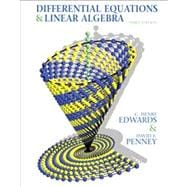# Differential Equations and Linear Algebra

• ISBN 13:

• ISBN 10:

## 0136054250

• Edition: 3rd
• Format: Hardcover
• Publisher: Pearson

Note: Not guaranteed to come with supplemental materials (access cards, study guides, lab manuals, CDs, etc.)

Extend Your Rental at Any Time

Sorry, this item is currently unavailable.

### Summary

Acclaimed authors Edwards and Penney combine core topics in elementary differential equations with those concepts and methods of elementary linear algebra needed for a contemporary combined introduction to differential equations and linear algebra. Known for its real-world applications and its blend of algebraic and geometric approaches, this book discusses mathematical modeling of real-world phenomena, with a fresh new computational and qualitative flavor evident throughout in figures, examples, problems, and applications. First-Order Differential Equations; Mathematical Models and Numerical Methods; Linear Systems and Matrices; Vector Spaces; Higher-Order Linear Differential Equations; Eigenvalues and Eigenvectors; Linear Systems of Differential Equations; Matrix Exponential Methods; Nonlinear Systems and Phenomena; Laplace Transform Methods; Power Series Methods. For future math majors, engineers, or scientists that have taken two or three semesters of Calculus.